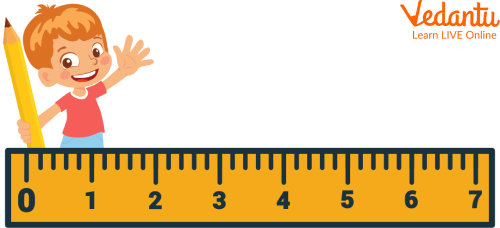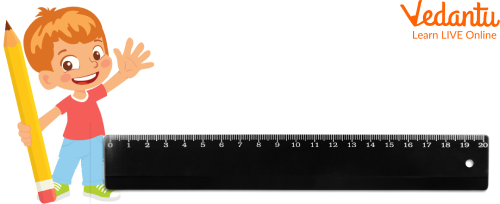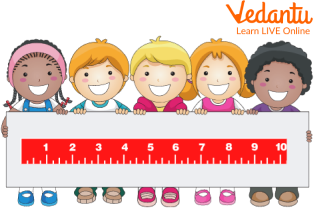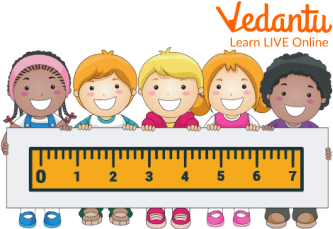Courses
Courses for Kids
Free study material
Free LIVE classes
More

# Meter ScaleLIVE
Join Vedantu’s FREE Mastercalss

## Overview of the Meter Scale

Have you ever thought about how to measure a meter? If not, then do not get impatient. This writing covers all the topics related to meter length, meter scale and measure meter. In the given article, children would gain an understanding of the concepts deeply such as how long is 1 meter and what is a meter long. The Meter scale is a very useful tool of geometry that helps students in comprehending higher levels of geometry. Now, let us start with the given topic.

## What is a Meter Scale?

A meter scale is defined as a mathematical set of tools which is generally used to calculate the length of any object or thing.Showing a Meter Scale

## What does the Meter Scale Measure?

The meter scale is used to measure meters of length of an object or thing. The SI unit of measuring a length using meter scale is meter, while its CGS unit is centimeter.

## How to Measure a Meter?

To measure using a meter scale, one must follow the given steps:

• Take the meter scale

• Put the meter scale on the starting point of the given length, object or thing

• Mark the reading on the scale, where the end of the length, object or thing points

Thus, the measured length is obtained.Showing the Plastic Meter Scale

## What is a Meter Long?

The length of an object is measured in the units of meter, centimeter, inches, yard or feet. A meter is about 100 centimeters long in length, i.e. 1 m = 100 cm. It is about 10 decimeters long, i.e. 1 m = 10 dm.

## Solved Examples

Q1. Explain the 10-decimeter length on a meter scale.

Ans: A 10 decimeter length on a meter scale describes 1 meter of length, i.e. 10 dm = 1m.Showing 10-Decimeter Length on Decimeter Scale

Q2. What happens when we subtract or add a meter with another meter?

Ans: When a meter is added or subtracted to another meter, the result of corresponding addition and subtraction is always in meters. We can’t add meters with centimeters directly. We need to keep both in the same measuring unit (either meter or centimeter).Showing a Wooden Meter Scale

## Practice Problems

Q1. What is the meaning of 1000 meters?

Ans: 1 km

Q2. Convert 18 m length into centimeters.

Ans: 1800 cm

Q3. Can a meter be converted to another unit of measurement such as centimeter, feet, inches etc?

Ans: Yes, a meter can be converted to the corresponding centimeter, feet, inches etc by multiplying or dividing with a constant power of 10. For example: 1 cm = \$\dfrac{1}{100}\$ m.

## Summary

Wrapping up here with the theory of meter scale and how to measure meters. It does describe how long 1 meter is? The language used in the description of the article is very easy and simple, which makes learning exciting and interesting. Some solved examples and practice problems are being given that need to be practiced by the students to gain better clarification of the topic. Feel open to implore your doubts by putting them down in the comments given below.

Last updated date: 28th Sep 2023
Total views: 94.5k
Views today: 2.94k

## FAQs on Meter Scale

1. What is the use of a compass?

A compass is a mathematical geometrical tool, which is used to draw various geometrical shapes and figures. It is also used to draw angles. It helps to find the midpoint and bisector of line segments.

2. What is used in making a meter scale?

A meter scale, in general,  is made of steel, plastic and wood. The meter scale made up of wood is called the wooden meter scale while the scale made up of steel is called the iron meter scale and the meter scale made up of plastic is called the plastic meter scale.

3. What is geometry?

Geometry is the branch of mathematics that deals with lines, shapes and figures. It is also defined as the spatial or visual arrangement of lines, curves, figures and shapes. Sometimes, it helps in finding the position of a geometric figure.